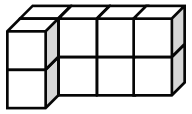### Home > CC1MN > Chapter 11 > Lesson 11.1.2 > Problem11-25

11-25.

Find the surface area and volume of the rectangular prism at right.Each edge of the above rectangular prism is $1$ unit.

Surface area is the total area of the surfaces of an object.

Volume is the space that an object occupies:
To find the volume, multiply the volume of one layer by the number of layers.

$10$ cubes make up this object.
How many sides act as a surface for the object and count towards the surface area?

$\text{Surface Area}=34\text{ square units}$
$\text{Volume} = 10 \text{ cubic units}$